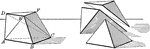### Triangular Pyramid Frustum

The frustum of a triangular pyramid is equivalent to the sum of three pyramids whose common altitude…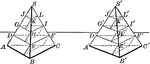### Equivalent Triangular Pyramids

Two triangular pyramids having equivalent bases and equal altitudes are equivalent.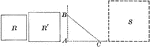### Square Constructed to be Equal to the Sum of Two Given Squares

Illustration of a how to construct a square equivalent to the sum of two given squares.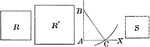### Square Constructed to be Equal to the Difference of Two Given Squares

Illustration of a how to construct a square equivalent to the difference of two given squares.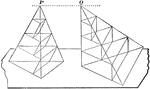### Equivalent Triangular Pyramids

Diagram used to prove the theorem: "Two triangular pyramids having equivalent bases and equal altitudes…